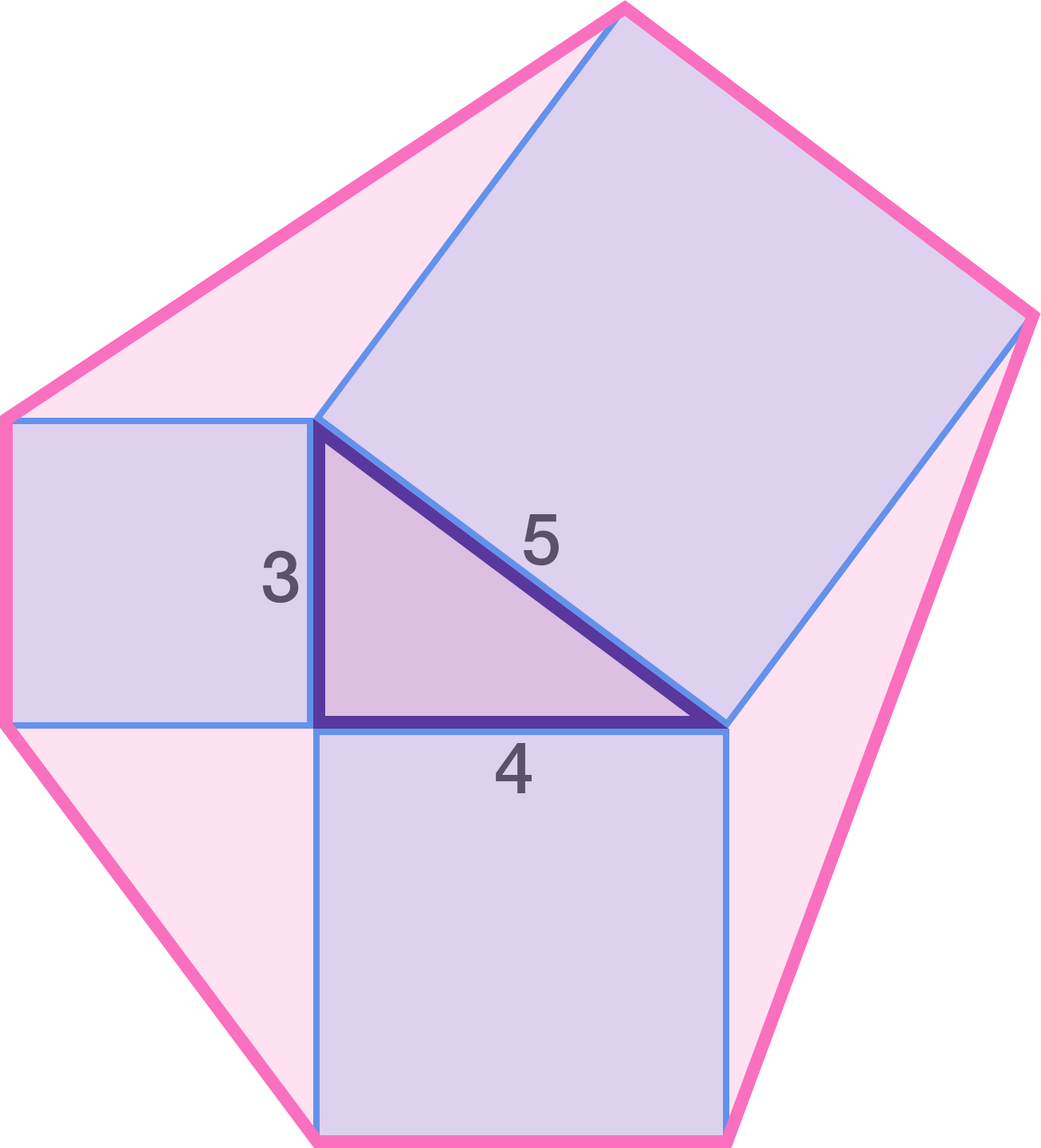# Triangle to a hexagon

Geometry Level 3Construct a square on each side of a triangle with side lengths 3, 4, and 5. Then, construct a hexagon by joining the vertices of the squares which are not vertices of the 3-4-5 triangle.

Find the area of this hexagon.

×